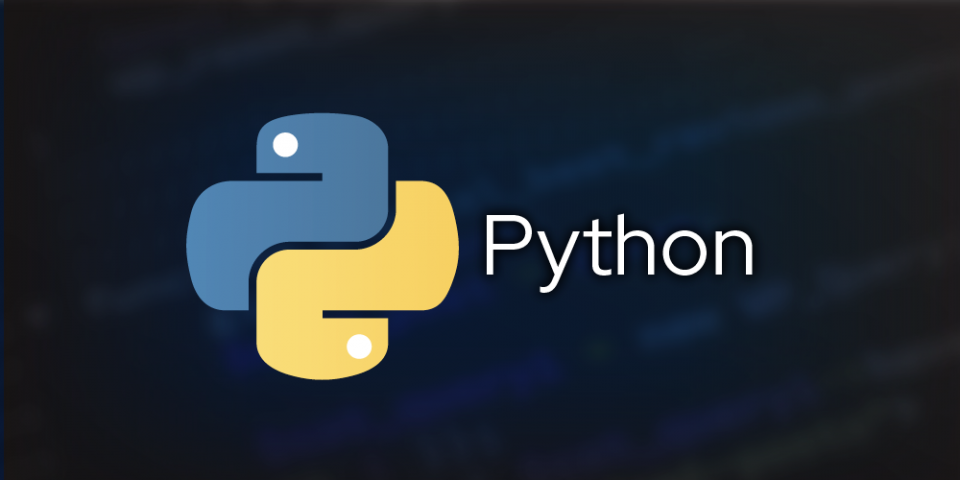I have been working on data analysis for almost three years, and there are some starters that I think are essential for every data analyst using the popular Pandas library for Python. If you often do data transformations in Pandas, you know how annoying it can be to search the web for basic information every time you get started with a new dataframe.

For me, one of those sore points is encoding text data. For some reason, I can never remember a good way to encode data when I need it. So, I decided to note down my three favorite ways of doing so. Let me know in the comments if you have any other alternatives.

## 1. Using the replace method with a dictionary

The `replace` method is great for manipulating column data in a Pandas dataframe. You can define a dictionary as an input argument for this method when converting a column of text data to integers. Let's take the simple dataframe called `data` with two columns, one text and one Boolean:

Index shouldihaveanothercoffee isitfridayyet
`0` `always` `True`
`1` `sure` `False`
`2` `definitely` `True`

You can convert the `shouldihaveanothercoffee` column to a numerical column using the replace method as follows:

``data["shouldihaveanothercoffee"].replace({"always":0, "sure":1, "definitely":2}, inplace=True)``

The following table shows the output from that statement:

Index shouldihaveanothercoffee
`0` `0`
`1` `1`
`2` `2`

## 2. Using the astype method

The `astype` method can convert data from one type to another. Boolean values to integers. Here, I'll show how you can use the method to convert a Boolean column `isitfridayyet` in the previously shown dataframe to Integer values (`True` being treated as `1` and `False` as `0`):

``data["isitfridayyet"] = data["isitfridayyet"].astype(int)``

The following table shows the output from that statement:

Index isitfridayyet
`0` `1`
`1` `0`
`2` `1`

## 3. Using the apply method

The `apply` method is another convenient method to handle data modifications for a data frame. You can use this method with explicit type conversion and the lambda function to convert data from Boolean to integer:

``data["isitfridayyet"] = data["isitfridayyet"].apply(lambda x: int(x)) ``

The following table shows the output from that statement:

Index isitfridayyet
`0` `1`
`1` `0`
`2` `1`